## Introduction

Topological states of matter, as quantum Hall systems or topological insulators, cannot be distinguished from ordinary matter by local measurements in the bulk of the material1,2,3,4. Instead, global measurements are required, revealing topological invariants as the Chern number. At the heart of topological materials are topologically protected edge states that occur at the intersection between regions of different topological order3,4,5,6,7. Ultracold atomic gases in optical lattices are promising new platforms for topological states of matter8,9,10,11,12,13, though the observation of edge states has so far been restricted in these systems to the state space imposed by the internal atomic structure14,15.

Here we report on the observation of an edge state between two topological distinct phases of an atomic physics system in real space using optical microscopy. An interface between two spatial regions of different topological order is realized in a one-dimensional optical lattice of spatially chirped amplitude. To reach this, a magnetic field gradient causes a spatial variation of the Raman detuning in an atomic rubidium three-level system and a corresponding spatial variation of the coupling between momentum eigenstates. This novel experimental technique realizes a cold atom system described by a Dirac equation with an inhomogeneous mass term closely related to the so called SSH model by Su, Schrieffer and Heeger16,17. The observed edge state is characterized by measuring the overlap to various initial states, revealing that this topological state has singlet nature in contrast to the other system eigenstates, which occur pairwise. We also determine the size of the energy gap to the adjacent eigenstate doublet.

## Results

### Background

Figure 1a shows a schematic of the spatial variation of the relevant band structure of rubidium atoms in the one-dimensional lattice. The lattice has a spatial periodicity of λ/4, where λ denotes the wavelength of the driving laser beams, with the corresponding potential being due to the dispersion of Raman transitions18,19. A magnetic field gradient imprints a spatially slowly varying lattice amplitude (in comparison with the scale of the lattice periodicity) via a modification of the Raman detuning. The achieved coupling K(z) between momentum eigenstates exp(±2ikz) determining the lattice amplitude, with k=2π/λ, varies spatially along the z axis. For not too large values of z the coupling follows K(z)=a·z, where a is a constant, and thus changes sign from negative to positive values of z. Here K(z) varies only slowly in space and it is therefore useful to discuss the bandstructure for fixed K(z)≈K(z0). At position z0=0, where the coupling K between momentum eigenstates vanishes, a crossing of Bloch bands occurs and the ordering of bands, indicated as |−〉 and |+〉, respectively, in Fig. 1a, is inverted. By continuous deformation the bands cannot be transformed into each other without closing the gap between bands. For such a situation a topologically protected edge state, localized around z=0, where the bands intersect, is expected20,21. Formally, this can be seen with a simple model describing the system near the crossing by a one-dimensional Dirac Hamiltonian22,23 with a spatially dependent effective mass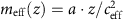: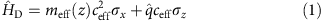where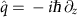is the momentum operator, σx and σz are Pauli matrices, and ceff=2ħk/m1.1 cm s−1 is an effective speed of light, with m as the rubidium atomic mass. The two-component Hamiltonian acts on spinors ψ(z)=(Ψ1(z),Ψ2(z)), with Ψ1 and Ψ2 corresponding to wavefunctions of atoms with momenta close to ±2ħk, respectively. The total wavefunction is given by ϕ(z)=Ψ1(z)e2ikz2(z)e−2ikz. The eigenenergies of the system are readily found to be E0=0 and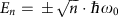for n>0, with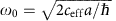. For quantum numbers n>0 the solution comes in pairs of opposite energies, while for n=0 there is only a singlet eigenstate, the topological edge state at the energetic position of the band crossing, which originates from the vanishing energy gap at the interface. The wavefunction of this state is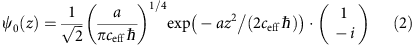which is the product of a Gaussian envelope and a coherent superposition of the two momentum eigenstates with a relative phase of π/2. As suggested by the discrete nature of the eigenenergies, all system eigenstates are bound states. Figure 1b shows the spatial variation of the probability density for the topological edge state (middle) and the doublet states with n=1, 2 and 3 (the corresponding plots on the top and bottom, respectively).

### Experimental realization

Our experiment starts by initially cooling a dilute cloud of rubidium atoms (87Rb) in the mF=−1 spin projection of the F=1 hyperfine component to Bose–Einstein condensation in a combined optical dipole and magnetic trap. A magnetic field gradient of magnitude m·g/(μB/2)30.5 G cm−1 compensates for the Earth’s gravitational acceleration. The cold atomic cloud is subsequently adiabatically expanded along the z axis to match the size of the topological edge state, of expected width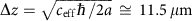, to obtain sufficient spatial overlap. The typical experimental width of the momentum distribution at this point is Δpz0.01ħk, corresponding to an effective kinetic temperature of 35 pK.

The lattice potential is realized using a rubidium atomic three-level configuration with two ground states of different spin projections and one spontaneously decaying excited state19 (Fig. 2a). To achieve a zero crossing of the coupling between bands, two four-photon potentials V1(z), V2(z) with opposite spatial variation of the coupling are superimposed. This is realized by choosing values of the Raman detuning δ1,2δ0μBΔB(z)/(2ħ) of opposite signs, where δ0 is a constant and ΔB(z)=B(z)−B(0)z·dB/dz with the spatially dependent magnetic field B(z) tuning the Zeeman splitting. We arrive at a lattice potential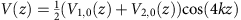with the envelopes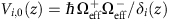, where Ωeff+ and Ωeff denote two-photon Rabi frequencies18. Expanding the amplitude of the lattice potential to first order around z=0 leads to Vi,0(z)=±V0+2a·z, with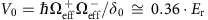, where Er=ħ2k2/(2m) denotes the recoil energy, and a=V0μB(dB/dz)/(4ħδ0)19.0·Er cm−1. The total lattice potential can be written in the simple form V(z)=2a·z cos (4kz), which experiences a zero crossing at z=0. Note that for z>0 (z<0) the maxima (minima) of the potential are located at integer multiples of λ/4 (Fig. 2b). This phase change is reflected in the inversion of band ordering. The dynamics of atoms in such a structure near the band crossing is described by the Dirac Hamiltonian of equation (1) with good accuracy (see Methods), as the width of the topological bound state Δz is two orders of magnitude larger than the lattice spacing.

### Characterization of the spatial variation of the band structure

In preparatory experiments we have characterized the band structure of the one-dimensional lattice focusing on the band-inversion on sign change of z. For this, the adiabatically expanded atomic cloud centred at different lateral positions z0 along the lattice beam axis (described by a wavefunction ϕa(zz0)) is transferred to the state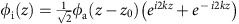via two simultaneously performed Bragg pulses24, described by the spinor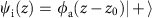with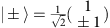(Fig. 1). To verify whether the cloud at the corresponding position overlaps with the upper or the lower band in the lattice, respectively, the band populations following activation of the lattice are determined. As shown in Fig. 3, for the chosen initial state we find that for z0<0 the loading is enhanced into the lower band, while for z0>0 most atoms are transferred into the upper band, and near z0=0 the curves cross. This experimentally verifies the expected spatial variation of the band structure, exhibiting a sign change of the coupling between momentum eigenstates at z0=0.

### Imaging cold atoms at the topological interface

We have next loaded atoms into the topological edge state. For this, using the Bragg pulses atoms are transferred to an initial state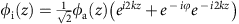with ϕ=π/2. The atomic wavepacket is centred at z=0, after which the lattice beams are activated. As the Bragg pulse increases the kinetic energy by more than two orders of magnitude, while the interaction energy remains largely unaffected, it turns out (see Methods) that interactions can be ignored for the subsequent time evolution.

Figure 4a (top) shows a series of atomic absorption images recorded after a variable holding time in the lattice along with a simulation (bottom). We observe that the atomic cloud remains trapped at the expected position of the atomic edge state. On the other hand, for a relative phase of ϕ=−π/2 no such trapping in the edge state is observed (Fig. 4b, top). This is in agreement with expectations, as when the initially prepared atomic wavepacket is π out of phase there is no overlap with the topological edge state. Instead, the wavepacket is split up into two spatially diverging paths. For larger times, the onset of an oscillation is visible, which during the experimentally accessible interaction times near 2 ms is only partially resolved. A fit yields a period of T=3.16(32)ms, see Methods. Such an oscillatory motion is also seen in the simulations (Fig. 4b, bottom). From theory we expect that for ϕ=π/2 the loading efficiency into the topological state is 95%, while for ϕ=−π/2 the wavepacket is mainly described by a coherent superposition of the two eigenstates of the first doublet with n=1, which beat with an oscillation period of T=π/ω0. Our experimental oscillation data allow us to determine the size of the splitting ω0/2π to 158(16) Hz, which is in good agreement with the expected value of 163 Hz, and gives a direct measurement for the size of the gap between the topologically protected edge state and the two energetically closest other system eigenstates. The observed lifetime near 2 ms is attributed to photon scattering from the Raman beams, an effect also assigned to be dominantly responsible for an observed residual expansion of the edge state visible in Fig. 4a (top). A further contribution to the observed residual expansion is a remaining mismatch of the initially prepared atomic wavepacket with the topological edge state, causing an admixture of eigenstates with larger values of n and correspondingly increased mode volume. This effect was accounted for in the model simulations shown in Fig. 4 (bottom), see Methods.

Figure 5a shows a series of absorption images recorded after a fixed time tint=1.7 ms for different values of the relative phase between momentum components of the initially prepared atomic wavepacket. Near a relative phase of ϕ=π/2 we again observe a compact atomic cloud, while for ϕ=−π/2 the cloud is split up into two components. A smooth variation between these extremes is visible for intermediate phase values. The corresponding variation of the total root mean squared (r.m.s.) width of the atomic cloud along the z axis versus the phase ϕ is shown in Fig. 5b.

We have next modified the overlap of the initial state and the topological state by preparing clouds with smaller initial size and correspondingly larger momentum spread by using different final values of the dipole trapping potential during adiabatic expansion. As described above, adiabatic expansion of the condensate cloud yields atomic ensembles with down to 0.01ħk momentum width, corresponding to effective temperatures in the pK regime. The dots in Fig. 5c show the relative variation of the total cloud width on the phase versus the momentum width of the atomic cloud. For a larger momentum spread of the initial wavepacket the observed phase dependency of the total cloud size reduces. This is attributed to the higher order system eigenstates with n>0, which are populated when loading with a wavepacket of larger momentum width than of the topological protected edge state. The experimental results are in agreement with a simulation (solid line). The inset of Fig. 5c shows the expected variation of the loading efficiency into the edge state on the momentum width of the cloud.

## Discussion

To conclude, an edge state at the spatial interface between two regions of different topological order has been observed by real-space imaging of the cold atomic cloud. Evidence for the successful population of this topological state has been obtained from (i) the phase dependence, (ii) the dependence of the initial atomic momentum width on the loading efficiency and (iii) the vibrational frequency of the lowest excited doublet modes agreeing with expectations.

For the future, it will be important to reveal the role of interactions on the topological edge state using, for example, Feshbach resonances for sensitive control, with prospects, including the simulation of interacting topological quantum matter25. Other perspectives include the realization of interacting relativistic wave equation predictions26, as well as novel topological Berry phase effects in phase space27.

## Methods

### Experimental apparatus and procedure

A schematic of the used experimental set-up is shown in Fig. 6. The experiment takes place within an ultrahigh vacuum chamber, which for the suppression of ac magnetic field noise is placed within a single layer μ-metal shielding with optical access for the cooling, trapping, detection and optical lattice beams. The magnetic field gradient required for the generation of the amplitude-chirped optical lattice and for the compensation of the Earth’s gravitational acceleration is produced by two pairs of identical coils in an anti-Helmholtz configuration oriented at an angle of 45° with respect to the vertical axis. This configuration in good approximation provides a two-dimensional (2D) quadrupole magnetic field of the form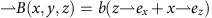(Fig. 6). The atomic cloud is placed ≈300 μm below the centre of the quadrupole field. At this position (with x≈0 and z>0), the field gradient for rubidium atoms (87Rb) in the F=1, mF=−1 component of the electronic ground state causes a vertically upwards directed Stern–Gerlach force. For a gradient b=mg/(μB|gF|)30.5 G cm−1, this compensates for the gravitational acceleration of rubidium atoms. Here gF=−1/2 denotes the gyromagnetic factor for the used F=1 hyperfine component of the electronic ground state. Experimentally, the gravitational force can be compensated to within 1 part in 104.

The used experimental set-up is a modified version of an apparatus used in earlier works19,24. The experiment proceeds by loading cold rubidium atoms collected in a magneto-optical trap into the dipole trapping potential provided by a focused beam derived from a CO2-laser operating near 10.6 μm wavelength. The atoms are evaporatively cooled by lowering the power of the mid-infrared trapping beam, which produces a Bose–Einstein condensate of 15,000 atoms in the F=1, mF=−1 component of the electronic ground state. The magnetic field gradient from the quadrupole field here allows for the production of a spin-polarized atomic cloud, and the quadrupole field provides additional confinement of the atomic cloud along the optical dipole beam axis. To enhance the overlap with the topological edge state, the condensate cloud is directly after production adiabatically expanded by further lowering the mid-infrared dipole trapping beam within a 10 s long ramp. During this expansion the effective trap size of the dipole trapping beam is effectively enlarged along the z axis by acousto-optic rapid modulation of its focal position (with ≈50 kHz modulation frequency). We arrive at an ensemble of ≈10,000 atoms of Δz11.5 μm r.m.s. spatial width along the lattice beam axis. The trapping frequency of the dipole potential along this axis at the end of this sequence is ωz/2π4 Hz. The r.m.s. momentum width of the trapped atomic cloud was determined to be Δpz≈0.01ħk along the lattice beam axis, using Bragg spectroscopy performed immediately after extinguishing the dipole trapping potential. This value corresponds to an effective kinetic temperature of 35 pK. Regarding the case of free atomic clouds, 2D matter wave lensing experiments with a rubidium Bose–Einstein condensate have achieved effective temperatures of 50 pK (ref. 28). For the data points with higher momentum spread shown in Fig. 5c, the adiabatic expansion of the condensate cloud proceeded to less low values of the trap potential, for which correspondingly also ωz at the end of the ramp was higher.

The optical radiation required to generate both the optical Bragg pulses and the optical lattice is derived from a single-mode high-power diode laser operating near 783.5 nm wavelength, 3.3 nm detuned to the red of the rubidium D2-line. Its emission is split into two, and each of the beams pass an acoustic-optical modulator used to imprint different optical frequency components. They are then guided through optical fibres to the vacuum chamber, where the beams are irradiated in a vertically oriented, counterpropagating geometry onto the cold atomic cloud.

Following the adiabatic expansion of the atomic cloud, the dipole trapping beam is extinguished and the atoms are irradiated with two simultaneously performed Bragg pulses of opposite direction of the momentum transfer, tuned to transfer atoms in a state ϕa(z) to the superposition, where ϕ denotes a variable relative phase. For more details on the Bragg-pulse technique, see ref. 24.

To synthesize the amplitude-chirped lattice we use lattice potentials realized in a Raman configuration (see Fig. 2a of the main text), because the local depth (and amplitude) of such a lattice can be selectively controlled with magnetic fields of moderate magnitude. The local field value tunes the local value of the Raman detuning via the Zeeman shift. Four-photon processes, driven by a beam of frequency ω and two counterpropagating fields of frequencies ωω and ω−Δω, respectively (Fig. 6), here induce a lattice potential with a λ/4 spatial periodicity18. The spatial periodicity of the four-photon lattice is a factor 2 smaller than the λ/2 periodicity of a usual standing wave lattice induced by two-photon processes. Doppler-sensitive four-photon Raman transitions couple the momentum states ei(2ħk+q)z/ħ and ei(−2ħk+q)z/ħ, respectively. Experimentally, the weak binding limit of the lattice potential is well fulfilled. For the two superimposed four-photon lattice potentials i=1, 2 with opposite spatial variation of the lattice depth we choose values for the frequency shift of Δω1=ωz+δ0 and Δω2=ωzδ0, respectively, where ωz/2π700 kHz denotes the size of the Zeeman splitting between adjacent spin projections at z=0 and δ0/2π200 kHz is the modulus of the Raman detuning at this spatial position. Both potentials are for atoms in the used mF=−1 spin projection of the F=1 hyperfine ground-state component. The two potentials V1(z) and V2(z) are generated using positive and negative two-photon detuning, respectively, and have an opposite spatial chirp of their corresponding lattice depth (as was shown in Fig. 2b of the main text). The potentials both have a spatial periodicity of λ/4, and by suitable choice of the phase values of the driving optical beams the potential maxima of say V1(z) are tuned to the position of the minima of V2(z), see Fig. 2b of the main text. The total potential experienced by the atoms V(z)=V1(z)+V2(z) can be written in the form V(z)=K(z)·2cos(4kz). With the quoted experimental parameters, the coupling K(z) is approximately linear in z in the experimentally relevant range (with the next term in the corresponding Taylor expansion ( z3) reaching about 25% of the linear term at positions z=±50 μm).

Both during the evaporative cooling and during the time that the lattice is switched on, the magnitude of the field gradient is set by the requirement that the Stern–Gerlach force compensates the Earth’s gravitational acceleration. However, besides imprinting the periodic lattice potential, the Raman coupling induced by the lattice beams cause a small admixture (≈2%) of the mF=0 to the mF=−1 Zeeman state. This reduces the effective magnetic moment, and thus the Stern–Gerlach force by a corresponding amount on activation of the lattice beams. For the data shown in Figs 4 and 5a,c, and the data points shown by the blue dots in Fig. 5b, this was compensated for by a small chirp of the lattice beams eigenfrequencies. This induces a counteracting inertial force in the atomic frame. For comparison, the green squares shown in Fig. 5b give the results for a second data set for which the applied magnetic field gradient was increased within 100 μs on activation of the lattice beams to compensate for the reduced magnetic moment of atoms. Here no chirp of the lattice beams frequencies was required. Within experimental uncertainties the results of both data sets agree. The latter data set exhibited enhanced statistical uncertainties in comparison with the first one, as understood from the switching process. The experimental lifetime of the atomic cloud in the lattice potential is mainly limited by photon scattering from the optical Raman radiation. A further contribution from interatomic scattering channels has been estimated to be negligible, see below.

Detection of the atomic cloud following the interaction with the lattice was performed after extinguishing the lattice beams by activating both repumping light (tuned to the F=1→F=2 component of the rubidium D2-line) and pulsing on a laser beam tuned to the F=2→F′=3 cycling component of this transition. This records a shadow image on a sCMOS camera (model Andor Zyla 5.5) to monitor the in site spatial distribution n(x,z) of the atomic cloud. For the used magnification of the imaging system, the size of one pixel of the camera corresponds to 0.985 μm in the object plane. The size of the observed atomic cloud images along the x axis (transversely to the axis of the lattice beams) is dominated by the spatial resolution of our imaging system, which in an independent measurement was determined to 4.8 μm r.m.s. spatial width.

### Analysis

To obtain the characteristic oscillation frequency ω0 from our experimental data, we have determined the (over x and y) integrated atomic density n(z)=∫ dz n(x,z), corresponding to one-dimensional profiles, from the measured absorption images. Figure 7a shows such profiles n(z) for atoms stored in the amplitude-chirped lattice for an interaction time t=1.7 ms both for ϕ=+π/2 (top) and ϕ=−π/2 (bottom), corresponding to the data shown in Fig. 4 in the main text for the corresponding interaction time. For the case of a phase ϕ=−π/2, we fitted a curve of the form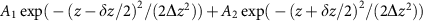to the corresponding profile, which allows us to determine a value for the spatial splitting δz between the two separating atomic clouds. Figure 7b shows the time evolution of the splitting δz for different holding times in the optical lattice, which clearly indicates the onset of an oscillatory motion. The value of the characteristic frequency ω0/2π≈158(16) Hz stated in the main text gives the obtained oscillation frequency of the sinusoidal fit. For the expected oscillation, see also Fig. 8c,d and the corresponding discussion below.

### Theoretical background

Assume that a rapidly oscillating potential V(z)=2K(z) cos(4kz) with a smooth envelop function K(z) (with K(z)=a·z in our experiment) scatters particles from momentum 2 to −2. Scattering to other momentum states is neglected in our treatment due to these states being energetically relatively far from resonance, see also ref. 19. Parametrizing the wavefunction by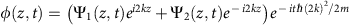, the Hamiltonian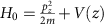can be mapped to a one-dimensional Dirac equation for the spinor ψ=(Ψ12) with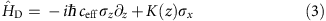where σi are Pauli matrices and we have linearized the dispersion around ±2 to obtain the effective speed of light ceff=2ħk/m. The Dirac equation possesses an emergent chiral symmetry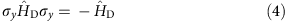According to Altland–Zirnbauer classification scheme29, systems with this symmetry belong to the class BDI. In one dimension such systems possess non-trivial topological states, which can be classified with an integer winding number3. Most importantly, states with K(z)>0 and K(z)<0 belong to two different topological classes, where the difference of winding numbers is 1. Topology enforces the existence of a bound state with energy 0 given by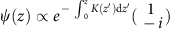for K(z→±∞)0 (ref. 30). All other states come in pairs with energy ±E due to the chiral symmetry (4). For K(z)=a·z the problem simplifies (and maps to a problem, exactly equivalent to the solution of the 2D Dirac equation in a magnetic field well known from graphene31). The observation that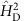maps (up to a spin-dependent shift) to a harmonic oscillator, motivates the introduction of bosonic raising and lowering operators, b, b, as used for a standard harmonic oscillator. Thus, the Dirac Hamiltonian (3) is written in the following form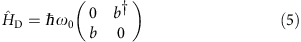where the matrix is given in the basis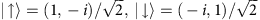and, and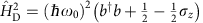. The eigenfunctions of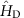are analytically described by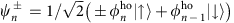for n≥1, and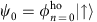for n=0, where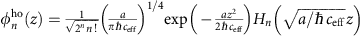are the eigenstates of a harmonic oscillator with oscillator length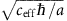and Hermite polynomials Hn(z). The spatial variation of the probability density for n≤3 was shown in Fig. 1b of the main text and the energy spectrum ofis given bywith a unique zero-energy eigenstate.

In Fig. 8a we show that for the experimental parameters the description in terms of the Dirac equation is valid with high precision for n20. In the figure we compare the eigenvalues of the Dirac equation HD with the full spectrum obtained by diagonalizing the original Hamiltonian H0.

### Interaction effects

Two types of interaction effects have to be considered for our set-up. First, interactions affect the initial state, that is, the shape of the wavefunction before the application of the Bragg pulse. Second, they modify the time evolution after the pulse. We will first show that the second effect is negligible while the first has to be taken into account.

To estimate the importance of interaction effects for the time evolution quantitatively, we consider a simplified one-dimensional situation, where we assume that in perpendicular direction only the lowest-energy states of a harmonic oscillator with frequencies ωx/2π=28.5 Hz and ωy/2π=10 Hz are occupied. This approximation actually overestimates interaction effects as it underestimates the size of the wavefunction in perpendicular directions. After projection onto degrees of freedom described by the Dirac spinor ψ=(Ψ1, Ψ2), one obtains a one-dimensional Dirac equation with local interaction and a corresponding Gross–Pitaevskii–Dirac equation, which takes the form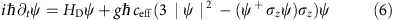with the dimensionless interaction strength given by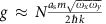, where we use the convention ∫|ψ|2=1. N≈104 is the number of particles, as≈100a0 is the scattering length of 87Rb, where a0 describes the Bohr radius, and ωx, ωy is the trapping frequency in the x and y direction, respectively. We obtain for our set-up g≈0.5. This factor parametrizes the ratio of the interaction energy and the kinetic energy. It is important to note that the dynamics of the condensate is therefore not dominated by interactions (in contrast to the initially prepared cloud, which had a kinetic energy, which was two orders of magnitude smaller). The dominant effect of g is a shift of all energies linear in g, but all observables studied in our experiment are affected only to quadratic order in g as a consequence of the chiral symmetry of HD. A quantitative analysis shows that all interaction effects turn out to be only on the per cent level and thus negligible within our present experimental resolution.

To study the interaction effects, we have solved both the stationary and the time-dependent Gross–Pitaevskii–Dirac equation and, furthermore, calculated the Bogoliubov spectrum. Figure 8b shows that the shape of the stationary solution of the Gross–Pitaevskii–Dirac equation for n=0 is almost unaffected by the interaction. Similarly, the density oscillations of the superposition of the doublet with n=1 are indistinguishable for g=0.5 and 0 (Fig. 8c,d). An important qualitative effect of interactions in the final state is that the system is intrinsically unstable. While for fermions Pauli blocking prohibits the decay of the topological surface state, the bosonic condensate wavefunction is not stable in the presence of interactions. As a consequence, the energies of the Bogoliubov spectrum describing the fluctuations around the stationary solution of the Gross–Pitaevskii–Dirac equation obtain imaginary contributions. An explicit calculation shows, however, that all decay rates are smaller than 1% of the typical oscillation frequency ω0.

While interaction effects thus do not play a role for the time evolution in our experiment, they do affect the initial state substantially. The main reason for this is that before the Bragg pulse is applied the kinetic energy is about two orders of magnitude smaller than after the pulse. Therefore, interaction effects dominate the initial, but not the final state. To gain a quantitative theoretical description of the experiment (lower panels in Fig. 4, green curve in Fig. 5c), we therefore proceed in the following way. To obtain the initial-state wavefunction (after adiabatic expansion, before the Bragg pulse and external lattice is switched on) we first calculate the static solution ψa(x,y,z) of the three-dimensional Gross–Pitaevskii equation using ref. 32. After the Bragg pulse (and after switching on of the lattice potential), the wavefunction is described by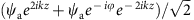. For the subsequent time evolution, we ignore interaction effects and use the non-interacting Dirac Hamiltonian (3) in combination with simple harmonic oscillator Hamiltonians for the perpendicular directions. The experiment determines the cloud from an absorption image in the xz plane. In the lower panel of Fig. 4 we therefore plot ∫dy1(x,y,z,t)|2+|Ψ2(x,y,z,t)|2 convolved with a Gaussian of r.m.s. width 4.8 μm to describe the effects of the finite experimental resolution. Note that the experimental resolution has little effect for the dynamics in z direction and mainly broadens the perpendicular direction. Rapidly oscillating interference fringes arising from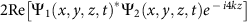cannot be resolved within the experimental resolution.

To obtain the green curve in Fig. 5c, we have repeated the above described calculations for several values of ωz, which gives us initial atomic distributions with different values of the momentum width. The quoted values for the momentum width are determined from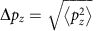in the initial state, the corresponding width of the final oscillating state from the value for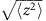, as obtained after interaction with the lattice.

### Data availability

The data that support the findings of this study are available from the corresponding authors on reasonable request.